Arithmetic
Arithmetic Progression
Associative Property
Averages
Brackets
Closure Property
Commutative Property
Conversion of Measurement Units
Cube Root
Decimal
Divisibility Principles
Equality
Exponents
Factors
Fractions
Fundamental Operations
H.C.F / G.C.D
Integers
L.C.M
Multiples
Multiplicative Identity
Multiplicative Inverse
Numbers
Percentages
Profit and Loss
Ratio and Proportion
Simple Interest
Square Root
Unitary Method
Algebra
Cartesian System
Order Relation
Polynomials
Probability
Standard Identities & their applications
Transpose
Geometry
Basic Geometrical Terms
Circle
Curves
Angles
Define Line, Line Segment and Rays
Non-Collinear Points
Parallelogram
Rectangle
Rhombus
Square
Three dimensional object
Trapezium
Triangle
Trigonometry
Trigonometry Ratios
Data-Handling
Arithmetic Mean
Frequency Distribution Table
Graphs
Median
Mode
Range

Videos
Solved Problems
Home >> Triangle >> Congruent Triangles >> Rules of Congruent Triangles >> ASA Rule of Congruent Triangles >>

## ASA Rule of Congruent Triangles

 SSS Rule of Congruent Triangles SAS Rule of Congruent Triangles ASA Rule of Congruent Triangles RHS Rule of Congruent Triangles

Before you study this topic; you are adviced to study

Define Congruence / Congruent
Congruent Triangles
Corresponding Parts of Congruent Triangles

ASA rule of Congruence illustrates that, if two angles and its included side are equal to the two corresponding sides and the included side of another triangle; then both the triangle are said to be congruent.

Example 1: In the following diagram: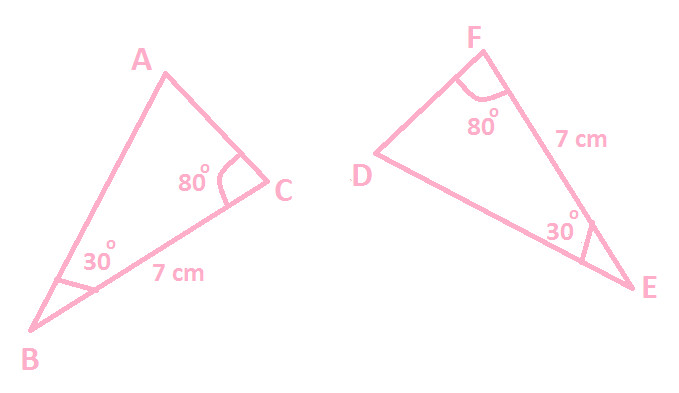Prove △ ABC ≅ △ DEF

Solution: In the given diagram:

BC = EF (7 cm each)
∠ B = ∠ E (30 Degree each)
∠ C= ∠ F (80 Degree each)

We can observe that:
Two angles (∠ B & ∠ C) of △ ABC are equal to corresponding two angles (∠ E & ∠ F) of △ DEF.
Side BC (included side to ∠ B & ∠ C) of ∆ ABC is equal to side EF (included side to ∠ E & ∠ F) of △ DEF
Therefore, from the above two observations, ASA congruence rules apply and we can say △ ABC and △ DEF are congruent.

And the corresponding relationships are as:
A ↔ D
B ↔ E
C ↔ F

Hence, △ ABC ≅ △ DEF

Example 2: In the following diagram: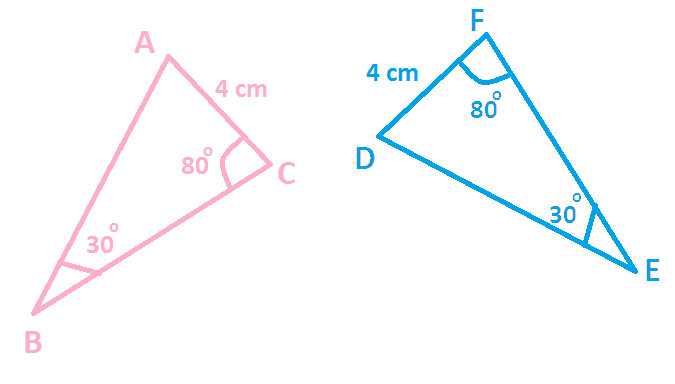Prove △ ABC ≅ △ DEF

Solution: In the given diagram:

AC = DF (7 cm each)
∠ B = ∠ E (30 Degree each)
∠ C= ∠ F (80 Degree each)

We can observe that:
Two angles (∠ B & ∠ C) of △ ABC are equal to corresponding two angles (∠ E & ∠ F) of △ DEF.
Side AC of △ ABC is equal to side DF of △ DEF
In the above 2nd observation, you can notice that sides AC & DF are not included side to ∠ B & ∠ C of △ ABC and to ∠ E & ∠ F of △ DEF respectively.
Therefore, SAS congruence rule fails to apply here and we get:
Hence, △ ABC not congruent △ DEF

Justification / Proof - ASA Congruence Rule

Now, let's justify or proof of ASA Rule of Congruence with the help of following three checks:

## Check 1:

There is a given triangle XYZ and measure of one of its ∠ X is equal to 60. You are asked to construct a triangle ABC congruent to triangle XYZ.
Can you do that ??
With measure of one of its angle is given i.e. 60, following triangles can be formed: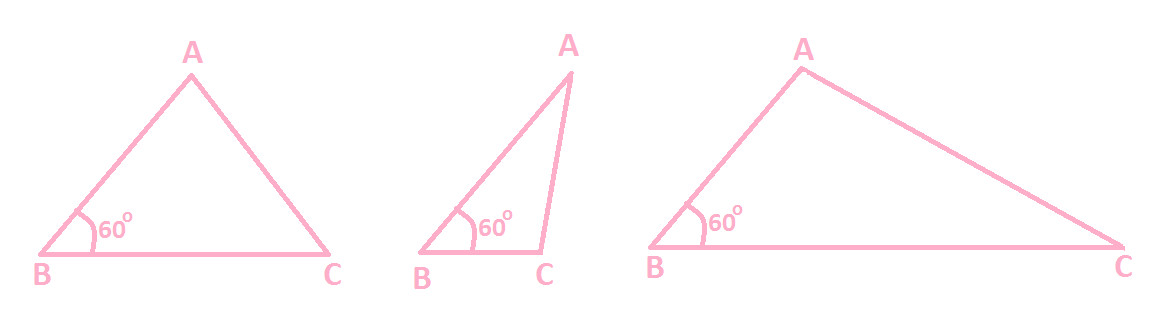From the above diagram of triangles, you can observe that given triangle XYZ can be any of the following and we are not sure which diagram of triangle is congruent to Triangle XYZ.

Hence, this confirms that two triangles cannot be congruent, if one angle of a triangle is equal to the corresponding one angle of another triangle.

## Check 2:

There is a given triangle XYZ , XY = 7cm & ∠ X = 60
Can you construct a triangle ABC congruent to triangle XYZ ?
With length of one side 7 cm & measure of one angle 60, we can construct many triangles:
(for instance)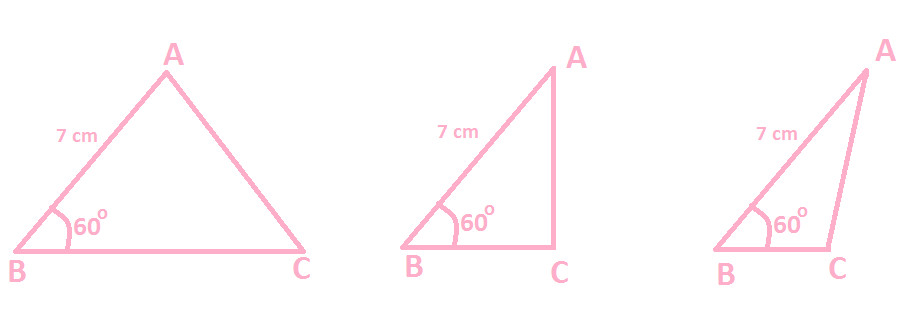From the above diagram of different triangles, you can observe that given triangle XYZ can be any of the following and we are not sure which diagram of Triangle ABC is congruent to Triangle XYZ.

Hence, this confirms that two triangles cannot be congruent, if one sides and one angle of a triangle are equal to the two corresponding sides and one corresponding angle of another triangle.

## Check 3:

There is a given triangle XYZ , where XY = 7 cm, ∠ X = 45 & ∠ Y = 60Can you construct a triangle ABC congruent to triangle XYZ ?
Let's try to construct a triangle ABC congruent to triangle XYZ, when measure of two angles and length of one side are given.
Steps of construction are as follows:

Step 1: Draw a line segment AB with length 5 cm, as shown in the below diagram: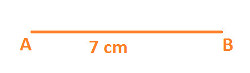Step 2: With the help of protractor and with A as center draw an angle of 45 and extend its arc to any convenient length, as shown below: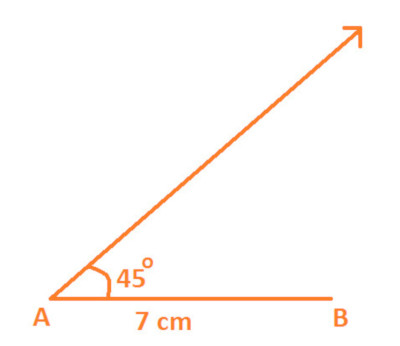Step 3: Now, again with the help of protractor and with B as center; draw an angles of 60 which intersects with arm of ∠ A at point C, as shown below: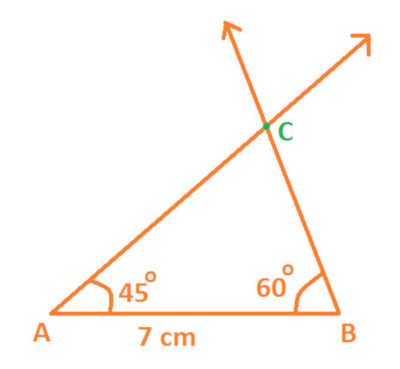Therefore resultant figure is a △ ABC with:
AB = 7 cm
∠ B = 60 °
∠ A = 45 °

Now you can see that:
AB XY (7 cm each)
∠ B ∠ Y (60 each)
∠ A ∠ X (45 each)
So, on the basis above correspondence we get:
△ ABC ≅ △ XYZ

Hence, this proves ASA rule of Congruence which says:
"If two angles and its included side are equal to the two corresponding sides and the included side of another triangle; then both the triangle are said to be congruent"

### Study More Solved Questions / Examples

 In the following diagram: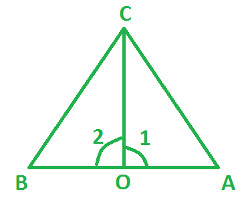CO is a bisector of ∠ AOB ∠ 1 = ∠ 2 Prove △ OBC ≅ △ OAC In the following diagram: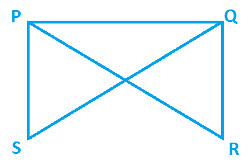∠ QPS = ∠ PQR ∠ PQS = ∠ QPR Prove △ SPQ ≅ △ RQP In the following diagram: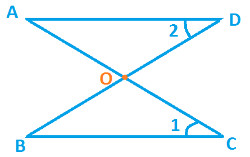DO = CO ∠ 1 = ∠ 2 Prove △ AOD ≅ △ BOC

Copyright@2022 Algebraden.com (Math, Algebra & Geometry tutorials for school and home education)